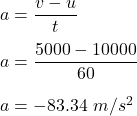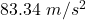## A satellite’s original velocity is 10,000 m/s. After 60 seconds it s going 5,000 m/s. What is the acceleration? Remember acceleration = (fin

Question

A satellite’s original velocity is 10,000 m/s. After 60 seconds it s going 5,000 m/s. What is the acceleration? Remember acceleration = (final speed – initial speed) ÷ time .

(p.s i think this is physics? i don’t know i just know its science so if anyone can help with this it would be greatly appreciated)

in progress 0
2 months 2021-07-30T04:39:27+00:00 1 Answers 0 views 0

1. Explanation:

Given that,

Orginal speed, u = 10,000 m/s

Final speed, v = 5,000 m/s

Time, t = 60 s

To find,

Acceleration of the satellite.

The acceleration of an object is equal to the change in its speed divided by time. So,So, the acceleration of the satellite isand it is deaccelerating.